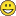# New to Qlik Sense

If you’re new to Qlik Sense, start with this Discussion Board and get up-to-speed quickly.

Announcements
Don't miss the upcoming Q&A with Qlik session on Qlik Application Automation on November 16th! REGISTER NOW
cancel
Showing results for
Did you mean:Partner

## How to flag on base of above and below criteria

Hello Friends,

I need a help in flagging data on base of above and below criteria in load script.

Step 1

IF any data contains  " | RR | BS | " then count as 1

Step 2

But IF any data contains  " | RR | AC | " with above Same Num & Ref number then count as -1

Step 3

But IF any data contains  " | RR | AC | " with below Same Num & Ref number then count as 0

I have attached sample file for your reference.

1 Solution

Accepted SolutionsPartner

Can u try this?

Table:

SubField(Data,'|',1) as Num,

SubField(Data,'|',SubStringCount(Data,'|')+1) as Ref,

SubField(Data,'|',1)&SubField(Data,'|',SubStringCount(Data,'|')+1) as Group,

Data

(ooxml, embedded labels, table is Sheet1) ;

NoConcatenate

Table1:

load *, RowNo() as row, if(Index(Data,' | RR | BS | ' )<>0,1

,

if(Index(Data,' | RR | AC | ' )<>0 and Group=previous(Group),-1

)

)

as Index1 Resident Table Order by Group;

drop table Table;

NoConcatenate

Final:

load * , if (len(Index1)=0 and len(Index2)=0,'',if(len(Index1)=0,Index2,Index1)) as Count ;

load *,if(Index(Data,' | RR | AC | ' )<>0 and Group=previous(Group),0)  as Index2

Resident Table1 order by row desc;

drop table Table1;

drop Fields row,Index1,Index2 from Final;

And see if it does the work?

4 RepliesPartner

Can u try this?

Table:

SubField(Data,'|',1) as Num,

SubField(Data,'|',SubStringCount(Data,'|')+1) as Ref,

SubField(Data,'|',1)&SubField(Data,'|',SubStringCount(Data,'|')+1) as Group,

Data

(ooxml, embedded labels, table is Sheet1) ;

NoConcatenate

Table1:

load *, RowNo() as row, if(Index(Data,' | RR | BS | ' )<>0,1

,

if(Index(Data,' | RR | AC | ' )<>0 and Group=previous(Group),-1

)

)

as Index1 Resident Table Order by Group;

drop table Table;

NoConcatenate

Final:

load * , if (len(Index1)=0 and len(Index2)=0,'',if(len(Index1)=0,Index2,Index1)) as Count ;

load *,if(Index(Data,' | RR | AC | ' )<>0 and Group=previous(Group),0)  as Index2

Resident Table1 order by row desc;

drop table Table1;

drop Fields row,Index1,Index2 from Final;

And see if it does the work?Partner
Author

Thank you 🙂 It worked.Partner

Well... that.. was... quick !Partner
Author

Yes, Thank you.

But I am stuck again. I need add 1 more different calculation. I have attached a file.

Please help me on it.Tags
Community Browser